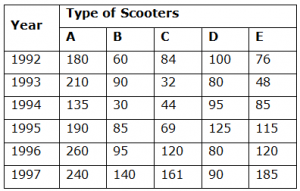## Data Interpretation For SBI PO : Set – 26

D.1-5) Study the table below and answer the following questions in reference to it.1) What is the price of a 7-minute DIAL DIRECT call to New York, NY, when you call in the evening?

a) \$1.56

b) \$1.94

c) \$1.65

d) \$1.74

e)

None of these

b)

First minute dial direct call to New York = .38 (evening)

Next 6 minute dial direct call to New York = 6*0.26 = 1.56

Total = .38 + 1.56 = 1.94

2) What is the difference in cost of a 7-minute DIAL DIRECT full rate call to New York, NY, and a 7-minute PERSON-TO-PERSON call to New York, NY?

a) \$1.55

b) \$3.00

c) \$4.55

d) \$4.10

e) None of these

b)

First minute = .62

Next 6 minute = .43 * 6 = 2.58

Total = 3.20

7 minute person to person call to NY = Dial Direct + 3 = 3.20 + 3 = 6.20

Difference Between them = 6.20 – 3.20 = 3

3) What is the price of a 12-minute OPERATOR ASSISTED Station-to-Station call to Miami, FL on a Tuesday at noon(Above 23 miles)?

a) \$5.48

b) \$7.03

c) \$8.45

d) \$7.53

e) None of these

b)

12 minute operator assisted station to station

For Miami FL, First minute =0.64

For next 11 minutes = 11 *.44 = 4.84

Above 12 miles = 1.55

Total = .64 + 4.84 + 1.55 = 7.03

4) What is the difference in price for a 9 minute DIAL DIRECT call to Los Angeles, CA, at 10:00 a.m. on a weekday  AND the same call made in the evening?

a) \$3.26

b) \$2.36

c) \$1.61

d) \$3.18

e) None of these

c)

9 minute Dial Direct call to Los Angeles LA,

In morning for first minute = .64

For next 8 minutes = 0.44 * 8 = 3.52

Total in morning = .64 + 3.52 = 4.16

In evening for first minute = .39

For next 8 minutes = .27 * 8 = 2.16

Total in evening = .39 + 2.16 = 2.55

Difference = 4.16 – 2.55 = 1.61

5) What is the cost of an 18 minute EVENING, OPERATOR ASSISTED â€“ STATION-TO-STATION call to New Orleans, LA (above 23miles)?

a) \$6.35

b) \$5.80

c) \$4.86

d) \$5.24

e) None of these

a)

Explanation

18 minute call to New Orleans, LA:-

First minute to New Orleans LA = .38

Next 17 minutes = 17 * .26 = 4.42

Above 23miles = 1.55

Total = 4.42 + .38 + 1.55 = 6.35

D.6-10) Study the following table and answer the questions given below it.

Production of different types of Scooters in a company6) In which of the following pairs of years was the total production of E-type scooter two lakhs?

a) 1993 & 1995

b) 1995 & 1997

c) 1994 & 1997

d) 1992 & 1994

e) None of these

e)

7) In which of the given years the total production of all types of scooters was minimum?

a) 1994

b) 1996

c) 1997

d) 1995

e) None of these

a)

In 1992 = 260+95+120+80+120=605

In 1993 = 210+90+32+80+48= 460

In 1994 = 135+30+44+95+85=389

In 1995 = 190+85+69+125+115= 584

In 1996 = 260+95+120+ 80+120 = 605

In 1997 = 240+140+161+90+185 = 816

So, The year 1994 has the minimum number of Production

8) In which of the given years was the production of B-type scooter close to its average production over given years?

a) 1995

b) 1994

c) 1993

d) 1992

e) None of these

a)

Average Production of Scooter ‘B’=60+90+30+85+95+140 = 500/3=83.3

Average value is 83.3 is close to  year 1995

9) In which year the production of D-type scooters was maximum?

a) 1993

b) 1996

c) 1995

d) 1997

e) 1992

c)

D type scooter Production in the year 1992 = 100

D type scooter production in the year 1993 = 80

D type scooter production in the year 1994 = 95

D type scooter production in the year 1995 = 125

D type scooter production in the year 1996 = 80

D type scooter production in the year 1997 = 90

Year 1995 has the maximum number of production

10) What was the average  production of A-type scooter in all the years  ?

a) 202.5

b) 150.5

c) 230

d) 400

e) 105